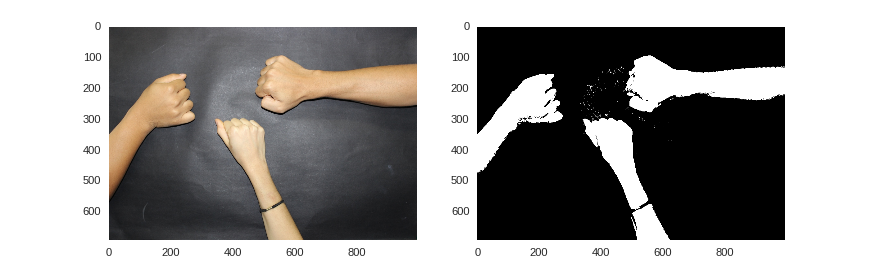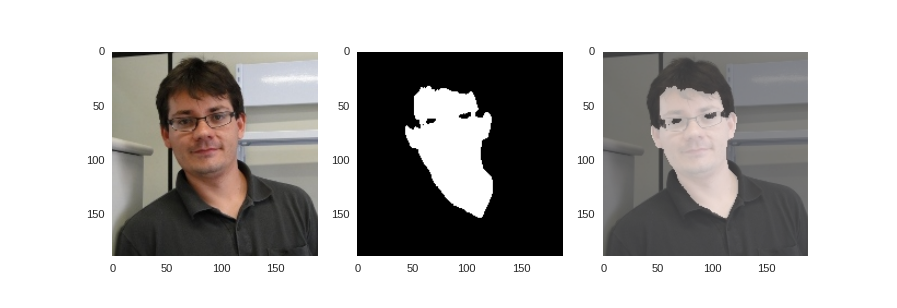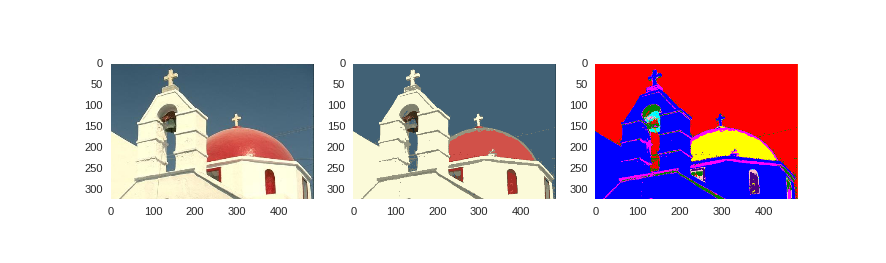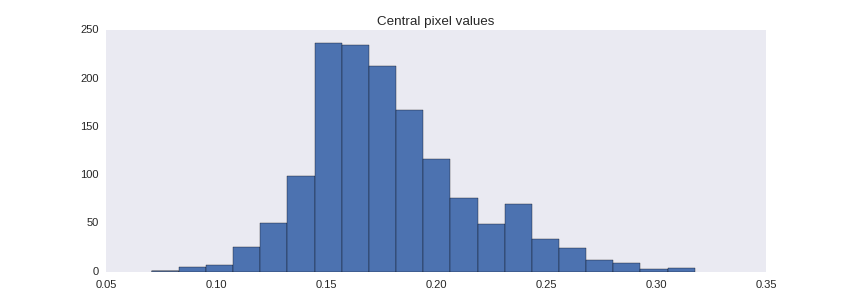# Scikit-learn¶

In [ ]:
import cv2

%matplotlib notebook
import numpy as np
from matplotlib import pyplot as plt
import seaborn as sns
sns.set_style("dark")

• Machine Learning (ML) is important for computer vision
• Learning
• Inference
• Scikit-learn is a module for machine learning algorithms
• Supervised learning
• Unsupervised learning
• Dimensionality reduction
• Parameter selection
• Cross-validation
• Development leadership by researchers at INRIA, France

The computer vision field relies strongly on machine learning methods and Bayesian inference. Machine learning provides the learning and inference tools for fitting and predicting the world state from images in several vision problems. The scikit-learn toolbox (or sklearn) is a machine learning package built on the SciPy Stack, developed by an international community of practitioners under the leadership of a team of researchers in INRIA, France. It provides tools for regression, classification, clustering, dimensionality reduction, parameter selection and cross-validation. Gaussian mixture models, decision trees, support vector machines, and Gaussian processes are a few examples of the methods available to date.

## Fit/Predict¶

• Scikit-learn's objects implements a fit/predict interface
• fit
• learning step (supervised or unsupervised)
• predict
• regression or classification
• The learned model can be stored using Python’s built-in persistence model, pickle

Sklearn is able to evaluate an estimator’s performance and parameters by cross-validation, optionally distributing the computation to several computer cores if necessary. The sklearn module implements machine learning algorithms as objects that provide a fit/predict interface. The fit method performs learning (supervised or unsupervised, according to the algorithm). The predict method performs regression or classification. The learned model can be saved for further usage by pickle, the Python’s built-in persistence model.

## Supervised learning in Sklearn¶

• Nearest Neighbors
• Support Vector Machines (SVM)
• Linear Support
• Radial Basis Function (RGB) kernel SVM
• Decision Trees
• Ensemble
• Random Forests
• Linear Discriminant Analysis
• Gaussian Processes

## Unsupervised learning in Sklearn¶

• Gaussian mixture models
• Clustering
• Affinity propagation
• Mean-shift
• Spectral clustering
• Hierarchical clustering
• DBSCAN
• Neural Networks (unsupervised)
• Restricted Boltzmann machines

This tutorial will not provide a full view of all methods available in sklearn. Instead, the basic usage will be illustrated by three examples on Naïve Bayes classification, mean-shift clustering and Gaussian Mixture models. For a broad and in-depth view on this module, the reader is referred to the sklearn on-line documentation, what is rich in descriptions, tutorials and code examples. Readers interest in machine learning and its applications in vision should refer to Bishop's and Prince's books.

## Example 6 - Skin detection using Naïve Bayes¶

In this example, Naïve Bayes classification is employed to detect pixels corresponding to human skin in images, based just in the pixel’s color measurements.

### Training data¶

• A $M \times N \times 3$ array, color a image in CIE Lab space
• A $M \times N$ binary mask representing reference classification
• Supervised learning
• L channel is discarded, avoiding lightness influence on skin detection

Let training be a $M \times N \times 3$ array representing a color training image in the CIE Lab color space, and mask a $M \times N$ binary array representing the manual classification skin/non-skin. The Gaussian fitting for Naïve Bayes classification will just use the chromaticity data (channels 1 and 2), avoiding lightness to influence on skin detection.

In :
training_bgr = cv2.imread('data/skin-training.jpg')
training_rgb = cv2.cvtColor(training_bgr, cv2.COLOR_BGR2RGB)
training = cv2.cvtColor(training_bgr, cv2.COLOR_BGR2LAB)
M, N, _ = training.shape

• The training image provides skin samples on a dark background
• Thresholding is employed to produce the binary mask
In :
mask = np.zeros((M,N))

In :
plt.subplot(1,2,1)
plt.imshow(training_rgb)
plt.subplot(1,2,2)Out:
<matplotlib.image.AxesImage at 0x7f49e942a550>
• Data is reshaped to a $MN$ vectors
• Each vector is 2-d, containing values for a anf b channels
• Slicing used to skip the L channel data

The data is composed by $MN$ 2d-vectors, easily extracted from the training image using reshaping and slicing.

In :
data = training.reshape(M*N, -1)[:,1:]
data

Out:
array([[128, 129],
[128, 129],
[128, 129],
...,
[127, 129],
[125, 134],
[123, 136]], dtype=uint8)

Similarly, the manual classification used in the learning step is represented as a binary $MN$ vector:

In :
target = mask.reshape(M*N)
target

Out:
array([ 0.,  0.,  0., ...,  0.,  0.,  0.])

#### Training (fitting)¶

• Gaussian Naïve Bayes is implemented by the GaussianNB object
• It presents a fit method for training

Sklearn provides a naive_bayes module containing a GaussianNB object that implements the supervised learning by the Gaussian Naïve Bayes method. As previously discussed, this object presents a fit method that performs the learning step:

In :
from sklearn.naive_bayes import GaussianNB
gnb = GaussianNB()
gnb.fit(data, target)

Out:
GaussianNB(priors=None)

#### Classification (prediction)¶

• Input image is converted to the CIE Lab color space
• Array is reshaped and sliced as the training data
• GaussianNB.predict is used for classification

Skin detection can be performed converting the input image to the Lab color space, and then reshaping and slicing in the same way as the training image. The predict method of GaussianNB performs the classification. The resulting classification vector can be reshaped to the original image dimensions for visualization.

In :
test_bgr = cv2.imread('data/thiago.jpg')
test_rgb = cv2.cvtColor(test_bgr, cv2.COLOR_BGR2RGB)
test = cv2.cvtColor(test_bgr, cv2.COLOR_BGR2LAB)
M_tst, N_tst, _ = test.shape

In :
data = test.reshape(M_tst * N_tst, -1)[:,1:]
skin_pred = gnb.predict(data)
S = skin_pred.reshape(M_tst, N_tst)

In :
plt.subplot(1,3,1)
plt.imshow(test_rgb)
plt.subplot(1,3,2)
plt.imshow(S, cmap=plt.cm.binary_r)
plt.subplot(1,3,3)
plt.imshow(test_rgb, alpha=0.6)
plt.imshow(S, cmap=plt.cm.binary_r, alpha=0.4)Out:
<matplotlib.image.AxesImage at 0x7f49c80e3550>

## Example 7 - Color segmentation using mean-shift clustering¶

In this example, the mean-shift algorithm is employed to perform color segmentation, grouping similar colors together (color quantization).

### Mean-shift¶

• The feature vectors are the pixels' color triplets in CIE Lab color space
• A $M \times N \times$ array is reshaped to $MN$ 3-d vectors

This clustering procedure relies in the Euclidean distance between the feature vectors, in this case the pixels’ color triplets. A perceptually uniform color space is more suitable to this task, once in such a space the Euclidean distances between triplets approximate the human perceptual differences. In this example, the Lab space is employed again. A view on the image is produced by reshaping, transforming the $M \times N$ array in a sequence of $M N$ 3-d vectors:

In :
I = cv2.imread('data/BSD-118035.jpg')
I_Lab = cv2.cvtColor(I, cv2.COLOR_BGR2LAB)
h, w, _ = I_Lab.shape
from sklearn.cluster import MeanShift, estimate_bandwidth
X = I_Lab.reshape(h*w, -1)
X

Out:
array([[ 33, 121, 120],
[ 33, 121, 120],
[ 33, 121, 120],
...,
[122, 122, 118],
[125, 122, 120],
[ 38, 122, 126]], dtype=uint8)
• Means-shift implementation in sklearn employs a flat kernel
• Such a kernel is defined by a bandwidth parameter
• Bandwidth can be automatically selected
• Sampling of inter-pixels color distances
• Euclidean distance in Lab approximates human perception
• A quantile is selected to pick the bandwidth value

The mean-shift implementation in sklearn employs a flat kernel defined by a bandwidth parameter. The bandwidth can be automatically selected by sampling the color distances be tween pixels in the input image and taking an arbitrary quantile selected by the user (larger quantiles generate bandwidths that produce fewer clusters). This procedure is implemented by the estimate_bandwidth function. Finally, the fit method is employed to perform the unsupervised learning:

In :
b = estimate_bandwidth(X, quantile=0.1, n_samples=2500)
ms = MeanShift(bandwidth=b, bin_seeding=True)
ms.fit(X)

Out:
MeanShift(bandwidth=12.296007814340724, bin_seeding=True, cluster_all=True,
min_bin_freq=1, n_jobs=1, seeds=None)

bin_seeding=True initializes the kernel locations to discretized version of points, where points are binned onto a grid whose coarseness corresponds to the bandwidth.

• ms.labels_ keeps the cluster identification for each pixel
• ms.cluster_centers_ stores the cluster centers
• The color quantization is performed attributing to each pixel the value the assigned cluster center

The labels_ attribute keeps the cluster attributed to each pixel, and the cluster_centers_ attribute stores the center value for each cluster. These centers are the quantized colors and will be employed on the visualization:

In :
S = np.zeros_like(I)
L = ms.labels_.reshape(h, w)
num_clusters = ms.cluster_centers_.shape
print num_clusters

for c in range(num_clusters):
S[L == c] = ms.cluster_centers_[c]

9

In :
plt.subplot(1,3,1)
plt.imshow(cv2.cvtColor(I, cv2.COLOR_BGR2RGB))
plt.subplot(1,3,2)
plt.imshow(cv2.cvtColor(S, cv2.COLOR_LAB2RGB))

from skimage.color import label2rgb
segments = label2rgb(L)
plt.subplot(1,3,3)
plt.imshow(segments)Out:
<matplotlib.image.AxesImage at 0x7f49b3651710>

In this example, just the pixel color was employed, resulting in color quantization. To perform spatial-color segmentation as proposed by Comaniciu and Meer, a multivariate kernel is needed. To the date, multivariate kernels are not available in sklearn’s mean-shift implementation.

## Example 8 - Background subtraction using Gaussian mixture models¶

The background of a video sequence is modeled using mixtures of Gaussians and further employed to classify people and objects as foreground.

Let $V$ be a $MN \times T$ array representing a video sequence composed by $T$ frames. Each frame is a $M \times N$ grayscale image. The background model is composed by M N mixtures of K multivariate Gaussians. Stauffer and Grimson proposed the use of Gaussian mixtures for background modeling because they are a simple and convenient way to represent multimodal distributions. Scenes in video sequences can present some sort of dynamic background, an issue commonly referred as the "waving trees" problem, and multimodal distributions are a better way to represent this variation.

• Each frame is a $M \times N$ grayscale image
• $V$ is a $MN \times T$ array, $T$ is time
In :
frames = !ls data/CAVIAR_LeftBag/*.jpg
# Let's find the frame dimensions, M x N
M, N = F.shape
T = len(frames)
M, N, T

Out:
(288, 384, 1439)

For each time $t$, insert pixels values in $V$, in the [0..1] interval:

In :
num_pixels = M * N
V = np.zeros((num_pixels, T), dtype=np.float)

for t, fname in enumerate(frames):
V[:,t] = np.array(F, dtype=float).reshape(-1)/255

V.shape

Out:
(110592, 1439)
In :
print V[num_pixels/2]

[ 0.15294118  0.16862745  0.14117647 ...,  0.21176471  0.21960784
0.19215686]

In :
hist = plt.hist(V[num_pixels/2], bins=20)
plt.title('Central pixel values')Out:
<matplotlib.text.Text at 0x7f49c8096d10>
In :
plt.subplot(1,2,1)
plt.imshow(V[:,0].reshape(M, N), cmap=plt.cm.gray)

plt.subplot(1,2,2)
plt.imshow(V[:,100].reshape(M, N), cmap=plt.cm.gray)